Know more about the Repeaters Batch Know more about the Repeaters Batch

# Center of Mass

## What is the Centre of Mass?

Centre of mass of a body or system of a particle is defined as, a point at which the whole of the mass of the body or all the masses of a system of particle appeared to be concentrated. In physics, we can say that the centre of mass is a point at the centre of the distribution of mass in space (also known as balance point) wherein the weighted relative position of the distributed mass has a sum of zero. In simple words, the centre of mass is a position that is relative to an object. We can say that it is the average position of all the parts of the system or it is the mean location of a distribution of mass in space. It is a point where force is usually applied that results in linear acceleration without any angular acceleration.

When we are studying the dynamics of the motion of the system of a particle as a whole, then we need not bother about the dynamics of individual particles of the system. But only focus on the dynamic of a unique point corresponding to that system.

Motion of this unique point is identical to the motion of a single particle whose mass is equal to the sum of all individual particles of the system and the resultant of all the forces exerted on all the particles of the system by surrounding bodies (or) action of a field of force is exerted directly to that particle. This point is called the centre of mass of the system of particles. The concept of centre of mass (COM) is useful in analyzing the complicated motion of the system of objects, particularly when two and more objects collide or an object explodes into fragments.

## Centre of Gravity

The Centre of gravity can be taken as the point through which the force of gravity acts on an object or system. It is basically the point around which the resultant torque due to gravity forces disappears. In cases where the gravitational field is assumed to be uniform, the centre of gravity and centre of mass will be the same. Sometimes these two terms – the centre of gravity and centre of mass are used interchangeably as they are often said to be at the same position or location.

### System of Particles

The term system of particles means a well-defined collection of a large number of particles that may or may not interact with each other or are connected to each other. They may be actual particles of rigid bodies in translational motion. The particle which interacts with each other apply force on each other.

$$\begin{array}{l}\text{The force of interaction}\ \overrightarrow{{{F}_{\hat{i}\,\hat{j}}}}\ \text{and}\ \overrightarrow{{{F}_{\hat{j}\,\hat{i}}}}\ \text{between a pair of}\ i^{th}\ \text{and}\ i^{th}\ \text{particle.}\end{array}$$
These forces of mutual interaction between the particle of the system are called the internal force of the system.

These internal forces always exist in pairs of equal magnitude and opposite directions. Other than internal forces, external forces may also act on all or some of the particles. Here the term external force means a force that is acting on any one particle, which is included in the system by some other body outside the system.

### What is a Rigid body?

In practice, we deal with extended bodies, which may be deformable or non-deformable (or) rigid. An extended body is also a system of an infinitely large number of particles having an infinitely small separation between them. When a body deforms, the separation between the distance between its particles and their relative locations changes. A rigid body is an extended object in which the separations and relative location of all of its constituent particles remain the same under all circumstances.

It is the average position of all the parts of the system, weighted according to their masses. For a simple rigid object which has a uniform density, the centre of mass is located at the centroid.

## Centre of Mass Videos

### Problem illustration on Centre of Mass## Determining the Center of Mass

If we were to experimentally determine a body’s centre of mass, we can make use of gravity forces on the body to do so. This can be done primarily because of the fact that the centre of mass is the same as the centre of gravity in the parallel gravity field near the earth’s surface. Moreover, the centre of mass of a body with an axis of symmetry and constant density will lie on this axis. Likewise, the centre of mass of a circular cylinder having constant density will have its centre of mass on the axis of the cylinder we talk about a spherically symmetric body of constant density then its COM is at the centre of the sphere. If we talk about it in a general context, for any symmetry of a body, its centre of mass will mostly be a fixed point of that symmetry.

### Centre of Mass Formula

We can extend the formula to a system of particles. The equation can be applied individually to each axis,

$$\begin{array}{l}X_{com}= \frac{∑_{i=0}^n~ m_i x_i }{M}\end{array}$$
$$\begin{array}{l}Y_{com}= \frac{∑_{i=0}^n~ m_i y_i}{M}\end{array}$$
$$\begin{array}{l}Z_{com} = \frac{∑_{i=0}^n~m_i z_i }{M}\end{array}$$

The above formula can be used if we have point objects. But we have to take a different approach if we have to find the center of mass of an extended object like a rod. We have to consider a differential mass and its position and then integrate it over the entire length.

$$\begin{array}{l}X_{com}=\frac{∫~x ~dm}{M}\end{array}$$
$$\begin{array}{l}Y_{com}=\frac{∫~y ~dm}{M} \end{array}$$
$$\begin{array}{l}Z_{com}=\frac{∫~z ~dm}{M} \end{array}$$

Suppose we have a rod as shown in the figure and we have to find its center of mass.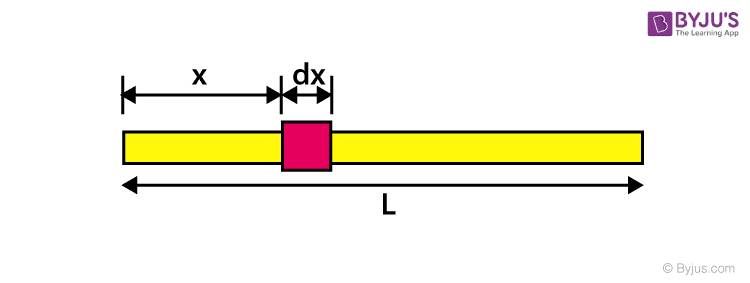Let the total mass of the rod be M, and the density is uniform. Also, we assume that the breadth of the rod is negligible i.e. the centre of mass lies on the x-axis. We consider a small dx at a distance from the origin. Therefore,

$$\begin{array}{l}dm = \frac{M}{l}~ dx\end{array}$$

Using the equation for finding center of mass,

$$\begin{array}{l}X_{com}=\frac{∫~\frac{M}{l}~ dx ~.x}{M}\end{array}$$
$$\begin{array}{l}X_{com}=\frac{∫~ dx ~.x}{l}\end{array}$$

Integrating it from 0 to l we get,

$$\begin{array}{l}X_{com}=\frac{l}{2}\end{array}$$

Using the above method we can find the center of mass for any geometrical shape. You can try out for a semi circular ring or a triangle. So if a force is applied to that extended object it can be assumed to act through the center of mass and hence, it can be converted to a point mass.

Summary Of Formulas:

 For point objects $$\begin{array}{l}Z_{com}= \frac{∑_{i=0}^n~m_i z_i }{M}\end{array}$$ For any geometrical shape $$\begin{array}{l}X_{com}=\frac{l}{2}\end{array}$$

#### Centre of mass of a body having continuous mass distribution

If the given object is not discrete and their distances are not specific, then centre of mass can be found by considering an infinitesimal element of mass (dm) at a distance x, y and z from the origin of the chosen coordinate system,

$$\begin{array}{l}{{x}_{cm}}=\frac{\int{xdm}}{\int{dm}}\end{array}$$
$$\begin{array}{l}{{y}_{cm}}=\frac{\int{ydm}}{\int{dm}}\end{array}$$
$$\begin{array}{l}{{z}_{cm}}=\frac{\int{zdm}}{\int{dm}}\end{array}$$

In vector form

$$\begin{array}{l}\overrightarrow{{{r}_{cm}}}=\frac{\int{\overrightarrow{r}dm}}{\int{dm}}\end{array}$$

#### Centre of mass for semi-circular ring of radius (R) and mass (M)

Solution: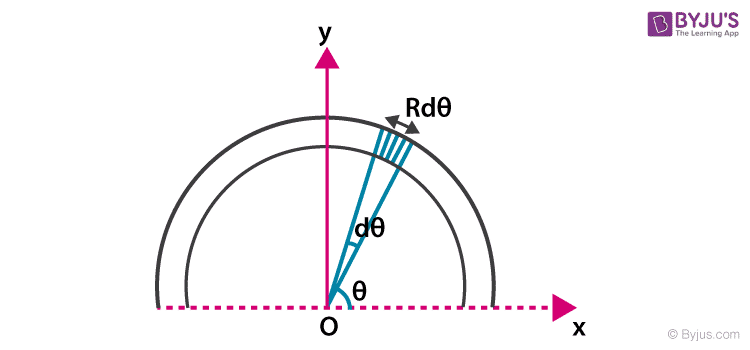Consider a differential element of length (dl) of the ring whose radius vector makes an angle θ with the x-axis. If the angle subtended by the length (dl) is dθ at the centre then

$$\begin{array}{l}dl=Rd\theta\end{array}$$

Then mass of the element is dm,

$$\begin{array}{l}dm=\lambda Rd\theta\end{array}$$

Since,

$$\begin{array}{l} \,\,\,{{x}_{cm}}=\frac{1}{M}\int{xdm}=\frac{1}{M}\int\limits_{0}^{\pi }{R\cos \theta \left( \lambda Rd\theta \right)}=0\end{array}$$

and

$$\begin{array}{l}{{y}_{cm}}=\frac{1}{M}\int\limits_{0}^{\pi }{\left( R\sin \theta \right)\left( \lambda Rd\theta \right)}\end{array}$$
$$\begin{array}{l}=\frac{\lambda {{R}^{2}}}{M}\int\limits_{0}^{\pi }{\sin \theta d\theta }=\frac{\lambda {{R}^{2}}}{\lambda \pi R}\left( -\cos \theta \right)_{0}^{\pi }\end{array}$$
$$\begin{array}{l}=\frac{2R}{\pi }\end{array}$$

Q. If linear mass density of a rod of length (L) lying along x-axis and origin at one end varies as

$$\begin{array}{l}\lambda =P+Qx\end{array}$$
where P and Q are constants, find the coordinates of the centre of mass.

Solution: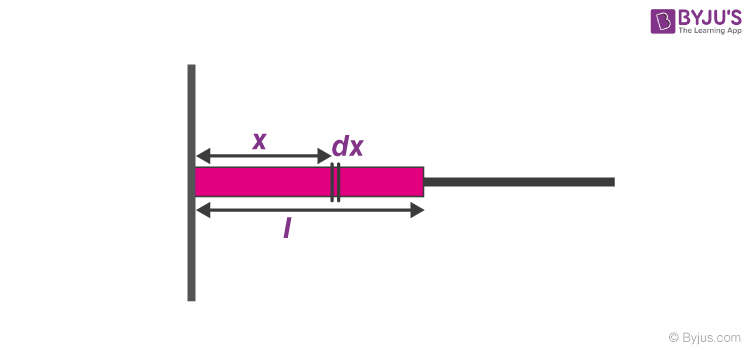The rod lies along x-axis hence

$$\begin{array}{l}{{y}_{cm}}=0\,\,\,and\,\,\,{{z}_{cm}}=0\end{array}$$

For

$$\begin{array}{l}{{x}_{cm}}:\end{array}$$

Consider a small element of (dx) at a distance x from one end of the rod

$$\begin{array}{l}{{x}_{cm}}=\frac{\int\limits_{0}^{L}{x\,dm}}{\int\limits_{0}^{L}{dm}}\end{array}$$

Mass of the element

$$\begin{array}{l}dm=\lambda dx=\left( P+Qx \right)dx\end{array}$$
$$\begin{array}{l}{{x}_{cm}}=\frac{\int\limits_{0}^{L}{x\left( P+Qx \right)dx}}{\int\limits_{0}^{L}{\left( P+Qx \right)dx}}=\frac{\frac{P{{L}^{2}}}{2}+\frac{Q{{L}^{3}}}{3}}{PL+\frac{Q{{L}^{2}}}{2}}\end{array}$$
$$\begin{array}{l}{{x}_{cm}}=\frac{L\left( 3P+2QL \right)}{3\left( 2P+QL \right)}\end{array}$$

and hence coordinates of the centre of mass are

$$\begin{array}{l}\left( \frac{L\left( 3P+2QL \right)}{3\left( 2P+QL \right)},0,0 \right)\end{array}$$

#### Centre of mass of a thin uniform disc of radius (R)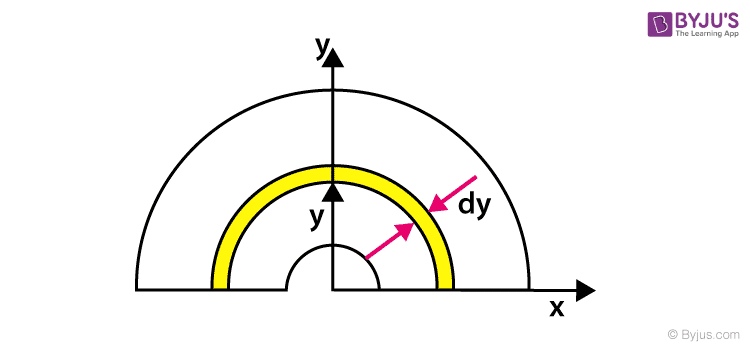Solution:

The disc is an analogy of a ring we are considering an elemental ring at y distance from origin and thickness dy.

As we know xcm = 0 (for a ring)

$$\begin{array}{l}{{y}_{cm}}=\frac{2R}{\pi }\ \text{(for a ring)}\end{array}$$

Now for disc

Disc is a combination of N number of elemental rings.

ycm for a disc is

$$\begin{array}{l}{{y}_{cm}}=\frac{1}{M}\int\limits_{0}^{R}{dm\,y}\end{array}$$
$$\begin{array}{l}dm=\frac{M}{A}da=\frac{M}{\frac{\pi {{R}^{2}}}{2}}\,\pi rdr\end{array}$$
$$\begin{array}{l}{{y}_{cm}}=\frac{1}{{M}}\int\limits_{0}^{R}{\left( \frac{{M}}{\frac{{\pi }{{R}^{2}}}{2}}\left( {\pi }rdr \right) \right)}\frac{2r}{\pi }\end{array}$$
$$\begin{array}{l}{{y}_{cm}}=\frac{4}{\pi {{R}^{2}}}\int\limits_{0}^{R}{{{r}^{2}}dr}\end{array}$$
$$\begin{array}{l}{{y}_{cm}}=\frac{4}{\pi {{R}^{2}}}\left[ \frac{{{R}^{3}}}{3} \right]\end{array}$$
$$\begin{array}{l}{{y}_{cm}}=\frac{4R}{3\pi }\end{array}$$

### System of Particles and Center of Mass

Till now we have dealt with the translational motion of rigid bodies where a rigid body is also treated as a particle. But when a rigid body undergoes rotation, all of its constituent particles do not move in an identical fashion. Still, we must treat it as a system of particles in which all the particles are rigidly connected to each other.

On the contrary, we may have particles or bodies not connected rigidly to each other but maybe interacting with each other through internal forces. Despite the complex motion of which a system of the particle is capable, there is a single point known as the centre of mass (or) mass centre whose translational motion is characteristic of the system.

#### Centre of Mass of a System of Particles

For a system consists of n particles, having masses

$$\begin{array}{l}{{m}_{1}},{{m}_{2}},{{m}_{3}},…{{m}_{n}}\end{array}$$
and their position vectors
$$\begin{array}{l}\overrightarrow{{{r}_{1}}},\overrightarrow{{{r}_{2}}},\overrightarrow{{{r}_{3}}},…\overrightarrow{{{r}_{n}}}\end{array}$$
respectively with respect to origin of the chosen reference frame, the position vector of centre of mass is rcm with respect to the origin is,

$$\begin{array}{l}\overrightarrow{{{r}_{cm}}}=\frac{{{m}_{1}}\overrightarrow{{{r}_{1}}}+{{m}_{2}}\overrightarrow{{{r}_{2}}}+{{m}_{3}}\overrightarrow{{{r}_{3}}}+…+{{m}_{n}}\overrightarrow{{{r}_{n}}}}{{{m}_{1}}+{{m}_{2}}+{{m}_{3}}+…+{{m}_{n}}}=\frac{{{m}_{1}}\overrightarrow{{{r}_{1}}}+{{m}_{2}}\overrightarrow{{{r}_{2}}}+{{m}_{3}}\overrightarrow{{{r}_{3}}}+…+{{m}_{n}}\overrightarrow{{{r}_{n}}}}{M}\end{array}$$

Here,

$$\begin{array}{l}{{m}_{1}}+{{m}_{2}}+{{m}_{3}}+…+{{m}_{n}}=M\end{array}$$

M is the total mass of the system,

Then,

$$\begin{array}{l}M\overrightarrow{{{r}_{cm}}}={{m}_{1}}\overrightarrow{{{r}_{1}}}+{{m}_{2}}\overrightarrow{{{r}_{2}}}+{{m}_{3}}\overrightarrow{{{r}_{3}}}+…+{{m}_{n}}\overrightarrow{{{r}_{n}}}….(1)\end{array}$$

Let an instant a system consists of large number of particles

$$\begin{array}{l}{{m}_{1}},{{m}_{2}},{{m}_{3}},…{{m}_{n}}\end{array}$$
and their positions vectors from the origin of chosen reference frame
$$\begin{array}{l}\overrightarrow{{{r}_{1}}},\overrightarrow{{{r}_{2}}},\overrightarrow{{{r}_{3}}},…\overrightarrow{{{r}_{n}}}\end{array}$$
changes as time passes, which indicates the system is in motion. At that instant particles of such system
$$\begin{array}{l}{{m}_{1}},{{m}_{2}},{{m}_{3}},…{{m}_{n}}\end{array}$$
located at
$$\begin{array}{l}\overrightarrow{{{r}_{1}}},\overrightarrow{{{r}_{2}}},\overrightarrow{{{r}_{3}}},…\overrightarrow{{{r}_{n}}}\end{array}$$
are moving with velocity
$$\begin{array}{l}\overrightarrow{{{v}_{1}}},\overrightarrow{{{v}_{2}}},\overrightarrow{{{v}_{3}}},…\overrightarrow{{{v}_{n}}}.\end{array}$$
$$\begin{array}{l}\text{So the mass center of the system located at}\ \overrightarrow{{{r}_{cm}}}\ \text{moves with velocity}\ \overrightarrow{{{v}_{cm}}}\end{array}$$
$$\begin{array}{l}M\overrightarrow{{{V}_{cm}}}={{m}_{1}}\overrightarrow{{{v}_{1}}}+{{m}_{2}}\overrightarrow{{{v}_{2}}}+{{m}_{3}}\overrightarrow{{{v}_{3}}}+…+{{m}_{n}}\overrightarrow{{{v}_{n}}}….(2)\end{array}$$

The mass centre motion represents the translational motion of the whole system. Sum of all particles linear momentum must be equal to linear momentum of the whole mass of the system due to translational motion of the centre of mass (or) mass centre.

We can also write the above eq (1) and (2) as follows

$$\begin{array}{l}M\overrightarrow{{{r}_{cm}}}=\sum{{{m}_{i}}\overrightarrow{{{r}_{i}}}}….(3)\end{array}$$
$$\begin{array}{l}M\overrightarrow{{{V}_{cm}}}=\sum{{{m}_{i}}\overrightarrow{{{V}_{i}}}}….(4)\end{array}$$

The above eq (3) location of center of mass of system of particles (or) discrete particles as follows

$$\begin{array}{l}\overrightarrow{{{r}_{cm}}}=\frac{\sum{{{m}_{i}}\overrightarrow{{{r}_{i}}}}}{M}….(5)\end{array}$$
$$\begin{array}{l}\text{In Cartesian coordinate system the position vector}\ \overrightarrow{{{r}_{cm}}}\ \text{ of center of mass in terms of components}\end{array}$$

$$\begin{array}{l}{{X}_{cm}}=\frac{\sum{{{m}_{i}}{{x}_{i}}}}{M}\end{array}$$
$$\begin{array}{l}{{Y}_{cm}}=\frac{\sum{{{m}_{i}}{{y}_{i}}}}{M}\end{array}$$
$$\begin{array}{l}{{Z}_{cm}}=\frac{\sum{{{m}_{i}}{{z}_{i}}}}{M}\end{array}$$

#### Two particle system

Let us consider a system consists of two particles of masses and their position vectors

$$\begin{array}{l}\overrightarrow{{{r}_{1}}}\ \text{and}\ \overrightarrow{{{r}_{2}}}\ \text{separation distance between them is d.}\end{array}$$
Position of centre of mass unaffected in the absence of external force.

$$\begin{array}{l}\text{Let us assume their center of mass located at}\ \overrightarrow{{{r}_{cm}}}\end{array}$$
, from the above eq (5)

$$\begin{array}{l}\overrightarrow{{{r}_{cm}}}=\frac{\sum{{{m}_{i}}\overrightarrow{{{r}_{i}}}}}{M}\end{array}$$
$$\begin{array}{l}\overrightarrow{{{r}_{cm}}}=\frac{{{m}_{1}}\overrightarrow{{{r}_{1}}}+{{m}_{2}}\overrightarrow{{{r}_{2}}}}{{{m}_{1}}+{{m}_{2}}}\end{array}$$

Its components in Cartesian coordinate system

$$\begin{array}{l}{{X}_{cm}}=\frac{{{m}_{1}}{{x}_{1}}+{{m}_{2}}{{x}_{2}}}{{{m}_{1}}+{{m}_{2}}}\end{array}$$
and
$$\begin{array}{l}{{Y}_{cm}}=\frac{{{m}_{1}}{{y}_{1}}+{{m}_{2}}{{y}_{2}}}{{{m}_{1}}+{{m}_{2}}}\end{array}$$
$$\begin{array}{l}\text{Let us assume origin at centre of mass(COM) vector}\ \overrightarrow{{{r}_{cm}}}.\end{array}$$
Both the particles lies on the x axis. Let the (COM) will also lie on the x axis.

$$\begin{array}{l}\text{Then}\ \overrightarrow{{{r}_{cm}}}\ \text{Vanishes.}\end{array}$$
$$\begin{array}{l}0={{m}_{1}}\overrightarrow{{{r}_{1}}}+{{m}_{2}}\overrightarrow{{{r}_{2}}}….(A)\end{array}$$

Either of the masses m1 and m2 cannot be negative. Then to satisfy the above relation

$$\begin{array}{l}\overrightarrow{{{r}_{1}}}\ and\ \overrightarrow{{{r}_{2}}}\ \text{must be in opposite direction.}\end{array}$$
$$\begin{array}{l}0={{m}_{1}}\left( -\overrightarrow{{{r}_{1}}} \right)+{{m}_{2}}\left( \overrightarrow{{{r}_{2}}} \right)\end{array}$$

Then

$$\begin{array}{l}{{m}_{1}}\overrightarrow{{{r}_{1}}}={{m}_{2}}\overrightarrow{{{r}_{2}}}\end{array}$$
$$\begin{array}{l}\frac{\overrightarrow{{{r}_{2}}}}{\overrightarrow{{{r}_{1}}}}=\frac{{{m}_{1}}}{{{m}_{2}}}….(B)\end{array}$$

From the eq (B)

$$\begin{array}{l}\overrightarrow{{{r}_{1}}}=\frac{{{m}_{2}}}{{{m}_{1}}}\overrightarrow{{{r}_{2}}}\,\,and\,\,\overrightarrow{{{r}_{2}}}=\frac{{{m}_{1}}}{{{m}_{2}}}\overrightarrow{{{r}_{1}}}\end{array}$$

As we know the separation distance between them is d,

$$\begin{array}{l}d=\overrightarrow{{{r}_{1}}}+\overrightarrow{{{r}_{2}}}\end{array}$$
$$\begin{array}{l}d=\frac{{{m}_{2}}}{{{m}_{1}}}\overrightarrow{{{r}_{2}}}+\overrightarrow{{{r}_{2}}}=\overrightarrow{{{r}_{2}}}\left( \frac{{{m}_{2}}}{{{m}_{1}}}+1 \right)\end{array}$$
$$\begin{array}{l}\overrightarrow{{{r}_{2}}}=d\frac{{{m}_{1}}}{{{m}_{1}}+{{m}_{2}}}\end{array}$$
similarly

$$\begin{array}{l}\overrightarrow{{{r}_{1}}}=d\frac{{{m}_{2}}}{{{m}_{1}}+{{m}_{2}}}\end{array}$$

This concludes that the centre of mass of the two-particle system lies between the two masses on the line joining them and divide the distance between them in the inverse ratio of their masses.

### Centre of Mass of the System with Cavity

If a part of a body is taken out, the remaining part of the body is considered to have

Existing mass = [{original mass (M)} + {-mass of the removed part (m)}]

Suppose there is a body of total mass m and a mass m1 is taken out from the body the remaining body will have mass (m – m1) and its mass center will be at coordinates

$$\begin{array}{l}{{X}_{cm}}=\frac{mx-{{m}_{1}}{{x}_{1}}}{m-{{m}_{1}}}:\end{array}$$
$$\begin{array}{l}{{Y}_{cm}}=\frac{my-{{m}_{1}}{{y}_{1}}}{m-{{m}_{1}}}\end{array}$$
and
$$\begin{array}{l}{{Z}_{cm}}=\frac{mz-{{m}_{1}}{{z}_{1}}}{m-{{m}_{1}}}\end{array}$$

Where (x, y and z) are coordinates of the centre of mass of the original body and

$$\begin{array}{l}\left( {{x}_{1}},{{y}_{1}}and\,{{z}_{1}} \right)\end{array}$$
are coordinates of centre of mass of portion taken out.

Centre of mass of an extended objects (Continuous distribution of mass):

An extended body is a collection of a large number of particles and closely located, their distances or not specific. and we assume the body as a continuous distribution of mass. Consider an infinitely small portion of mass dm of the body which is known as a mass element. The position vector of the centre of mass of such an object is given by,

$$\begin{array}{l}\overrightarrow{{{r}_{cm}}}=\frac{\int{\overrightarrow{r}dm}}{M}….(6)\end{array}$$

Its components in the Cartesian coordinate system are as follows,

$$\begin{array}{l}{{X}_{cm}}=\frac{\int{xdm}}{M}\end{array}$$
$$\begin{array}{l}{{Y}_{cm}}=\frac{\int{ydm}}{M}\end{array}$$
and
$$\begin{array}{l}{{Z}_{cm}}=\frac{\int{zdm}}{M}\end{array}$$

### Motion of the Centre of Mass

A system consists of n number of particles having masses

$$\begin{array}{l}{{m}_{1}},{{m}_{2}},{{m}_{3}},…{{m}_{n}}\end{array}$$
and total mass of the system is M. From the definition of centre of mass,

$$\begin{array}{l}M\overrightarrow{{{r}_{cm}}}={{m}_{1}}\overrightarrow{{{r}_{1}}}+{{m}_{2}}\overrightarrow{{{r}_{2}}}+{{m}_{3}}\overrightarrow{{{r}_{3}}}+…+{{m}_{n}}\overrightarrow{{{r}_{n}}}….(1)\end{array}$$

If the mass of each particle of the system remains constant with time, for this system of particles with fixed mass, differentiating the above eq with respect to time we get

$$\begin{array}{l}M\frac{d\overrightarrow{{{r}_{cm}}}}{dt}={{m}_{1}}\frac{d\overrightarrow{{{r}_{1}}}}{dt}+{{m}_{2}}\frac{d\overrightarrow{{{r}_{2}}}}{dt}+{{m}_{3}}\frac{d\overrightarrow{{{r}_{3}}}}{dt}+…+{{m}_{n}}\frac{d\overrightarrow{{{r}_{n}}}}{dt}….(2)\end{array}$$
$$\begin{array}{l}M\overrightarrow{{{V}_{cm}}}={{m}_{1}}\overrightarrow{{{v}_{1}}}+{{m}_{2}}\overrightarrow{{{v}_{2}}}+{{m}_{3}}\overrightarrow{{{v}_{3}}}+…+{{m}_{n}}\overrightarrow{{{v}_{n}}}….(3)\end{array}$$

Here,

$$\begin{array}{l}\overrightarrow{{{r}_{1}}},\overrightarrow{{{r}_{2}}},\overrightarrow{{{r}_{3}}},…\overrightarrow{{{r}_{n}}}\ \text{are position vectors of individual particles 1, 2 and 3 … n}\end{array}$$
$$\begin{array}{l}\overrightarrow{{{v}_{1}}},\overrightarrow{{{v}_{2}}},\overrightarrow{{{v}_{3}}},…\overrightarrow{{{v}_{n}}}\ \text{are velocity vectors of individual particles 1, 2 and 3 … n}\end{array}$$
$$\begin{array}{l}\overrightarrow{{{r}_{cm}}}\ and\ \overrightarrow{{{V}_{cm}}}\ \text{are position vector and velocity vector of centre of mass.}\end{array}$$

Differentiating the velocity expression we will get,

$$\begin{array}{l}M\frac{d\overrightarrow{{{v}_{cm}}}}{dt}={{m}_{1}}\frac{d\overrightarrow{{{v}_{1}}}}{dt}+{{m}_{2}}\frac{d\overrightarrow{{{v}_{2}}}}{dt}+{{m}_{3}}\frac{d\overrightarrow{{{v}_{3}}}}{dt}+…+{{m}_{n}}\frac{d\overrightarrow{{{v}_{n}}}}{dt}….(4)\end{array}$$
$$\begin{array}{l}M\overrightarrow{{{a}_{cm}}}={{m}_{1}}\overrightarrow{{{a}_{1}}}+{{m}_{2}}\overrightarrow{{{a}_{2}}}+{{m}_{3}}\overrightarrow{{{a}_{3}}}+…+{{m}_{n}}\overrightarrow{{{a}_{n}}}….(5)\end{array}$$

Where

$$\begin{array}{l}\text{Where}\ \overrightarrow{{{a}_{1}}},\overrightarrow{{{a}_{2}}},\overrightarrow{{{a}_{3}}},…\overrightarrow{{{a}_{n}}}\ \text{are velocity vectors of individual particles 1, 2 and 3 … n and}\end{array}$$
$$\begin{array}{l}\overrightarrow{{{a}_{cm}}}\ \text{is the acceleration of centre of mass, from Newton’s second law of motion,}\end{array}$$

The force Fi acting on the ith particle is given by,

$$\begin{array}{l}\overrightarrow{{{F}_{i}}}={{m}_{i}}\overrightarrow{{{a}_{i}}}\end{array}$$

The above eq (5) can be written as,

$$\begin{array}{l}M\overrightarrow{{{a}_{cm}}}=\overrightarrow{{{F}_{1}}}+\overrightarrow{{{F}_{2}}}+\overrightarrow{{{F}_{3}}}+…+\overrightarrow{{{F}_{n}}}=\overrightarrow{{{F}_{{internal}}}}+\overrightarrow{{{F}_{external}}}…..\ \ ….(6)\end{array}$$

Where F1, F2, F3 and Fn are the forces acting on the individual particles 1, 2 and 3 … n of the system

The internal forces are the forces exerted by the particles of the system on each other, however, from newton’s third law, these internal forces occur as pairs of equal magnitude and opposite direction. So their net sum is zero. Then the above eq (6) becomes

$$\begin{array}{l}M\overrightarrow{{{a}_{cm}}}=\overrightarrow{{{F}_{external}}}…………(7)\end{array}$$

Eq (7) states that the COM of the system of particles behaves like all the mass of the system were concentrated there and the resultant of all the external forces acting on all the particles of the system was applied on COM.

### Problems on Center of Mass

1. Two identical rods each of mass (m) and length (L) are connected as shown in the figure. Locate the centre of mass of the system.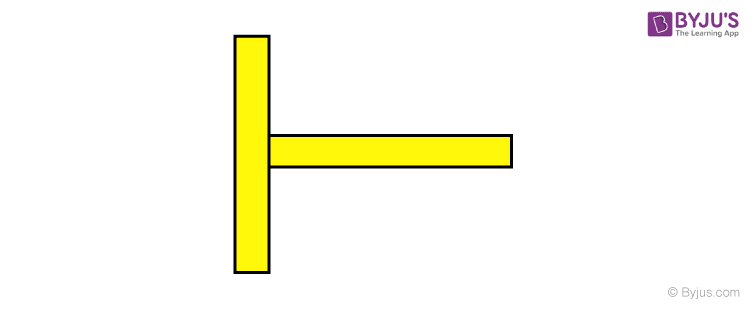Solution:

This system is symmetrical about x-axis hence we need to find Here we take coordinates of CM of rods.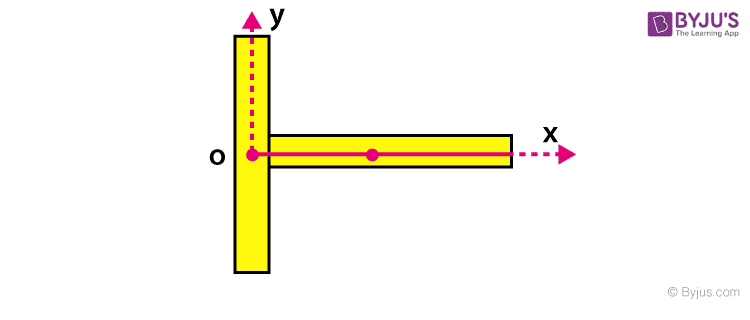M1 = M2 = M

L1 = L2 = L

Where M1, M2 and L1, L2 are mass and length of Rod 1 and Rod 2

$$\begin{array}{l}{{x}_{cm}}=\frac{{{m}_{1}}{{x}_{1}}+{{m}_{2}}{{x}_{2}}}{{{m}_{1}}+{{m}_{2}}}=\frac{M\left( 0 \right)+M\left( \frac{L}{2} \right)}{M+M}\end{array}$$

2. A system consisting of two objects has a total momentum of (16 kgm/sec)i and its centre of mass has the velocity of (2 m/s)i. One of the objects has a mass 5 kg and velocity (1.6 m/s)i. The mass and velocity of the other objects?

Given

Total momentum = (16 kgm/sec)i

Velocity of the center of mass = (2 m/s)i

Mass of one object=5 kg

Velocity of this object=(1.6 m/s)i

To calculate m and ν?

Solution:

Total momentum =Total mass X× velocity of the center of mass

(16 kgm/sec)i = (m+ 5) × (2 m/s)i

m =3 kg

Now Vcm=(m1v1+m2v2)/(m1+m2)

2=(5 ×1.6 + 3 × v2)/(5+3)

2 = (8 +  3v2) /8

16 = 8 + 3v2

3v2 = 8

v2 = (2.67 m/s)i

## Centre of Mass and Collision – Important Questions## Centre of Mass and Collision – Important Topics## Frequently Asked Questions on Centre of Mass

### Is it necessary that the centre of mass should always lie inside the body?

No, it may lie outside the body.

### What is the position of the centre of mass for a uniform rod, circular ring, circular disc and sphere?

In all cases, it is at the centre of the body.

### Can the geometrical centre and centre of mass of a body coincide?

Yes. The geometrical centre and centre of mass of spheres, circular rings, circular discs, cubes, cylinders coincide.

Test your Knowledge on Center Of Mass